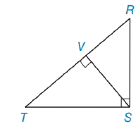Chapter 5.4, Problem 1EElementary Geometry For College St...

7th Edition
Alexander + 2 others
ISBN: 9781337614085

Solutions

Chapter
SectionElementary Geometry For College St...

7th Edition
Alexander + 2 others
ISBN: 9781337614085
Textbook Problem

By naming the vertices in order, state three different triangles that are similar to each other.Exercises 1-6

To determine

To Find:

The proof of similarity of triangles.

Explanation

Given figure,

From the figure,

ΔRST is a right triangle with right angle S, and SV¯ is the altitude to TR¯.

 Proof Statements 1 SV¯ is the altitude to TR¯. Given 2 SV¯⊥TR¯ An altitude is a segment from a vertex and perpendicular the opposite side. 3 ∠RVS and ∠SVT are right angles Angles forms by perpendicular lines are perpendicular 4 ∠S is a right angle Given 5 ∠RVS≅∠SVT≅∠S Right angles are congruent 6</

Still sussing out bartleby?

Check out a sample textbook solution.

See a sample solution

The Solution to Your Study Problems

Bartleby provides explanations to thousands of textbook problems written by our experts, many with advanced degrees!

Get Started